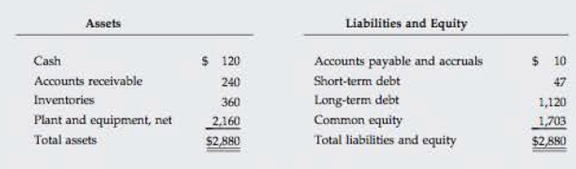Chapter 10, Problem 9PFundamentals of Financial Manageme...

15th Edition
Eugene F. Brigham + 1 other
ISBN: 9781337395250

Solutions

Chapter
SectionFundamentals of Financial Manageme...

15th Edition
Eugene F. Brigham + 1 other
ISBN: 9781337395250
Textbook Problem

WACC The Paulson Company’s year-end balance sheet is shown below. Its cost of common equity is 14%, its before-tax cost of debt is 10%, and its marginal tax rate is 40%. Assume that the firm’s long-term debt sells at par value. The firm’s total debt, which is the sum of the company’s short-term debt and long-term debt, equals $1,167. The firm has 576 shares of common stock outstanding that sell for$4.00 per share. Calculate Paulson’s WACC using market-value weights.Summary Introduction

To calculate: P Company’s WACC using market value weights.

Introduction:

Weighted Average Cost of Capital:

It is the weighted average cost of capital of all the sources through which a firm finances its capital. It is the rate that a company will pay to all for raising finance. It can be termed as the firm’s cost of capital. The company raises money through various sources such as common stock and preference share debt. The WACC is computed by taking the relative weight of each item of the capital structure.

Explanation

Given information:

Before-tax cost of debt is 10% or 0.10.

Cost of equity is 14% or 0.14.

Calculated info,

Weight of debt is 0.3271.

Weight of equity is 0.6728.

The company does not have any preferred stock the WACC can be calculated as

WACC=Wdrd(1t)+Wcrs

Where

• Wd is the weight of the debt.
• Wc is the weight of the equity.
• rd is cost of the debt.
• rc is the cost of the equity.

Substitute 0.3271 for Wd , 0.10 for rd 0.14 for rc and 0.6728 for Wc in above formula.

=0.3271×0.10(10.40)+0.6728×0.14=0

Still sussing out bartleby?

Check out a sample textbook solution.

See a sample solution

The Solution to Your Study Problems

Bartleby provides explanations to thousands of textbook problems written by our experts, many with advanced degrees!

Get Started

Why must researchers document their sources meticuousIy?

Essentials of Business Communication (MindTap Course List)

How does a cost-efficient capital market help reduce the prices of goods and services?

Fundamentals of Financial Management, Concise Edition (with Thomson ONE - Business School Edition, 1 term (6 months) Printed Access Card) (MindTap Course List)

What are the three major parts of a T account?

College Accounting, Chapters 1-27 (New in Accounting from Heintz and Parry)